## Tutorial: Calculate the Topographic Wetness Index from a DTM

### 3. Calculate Slope in Degrees and in Radians

In order to calculate TWI we need the slope in radians. With the raster analysis tools we can calculate the slope in degrees and percentages. So we're first going to calculate the slope in degrees and then convert the values to radians.

1. In the main menu go to Raster | Analysis | Slope...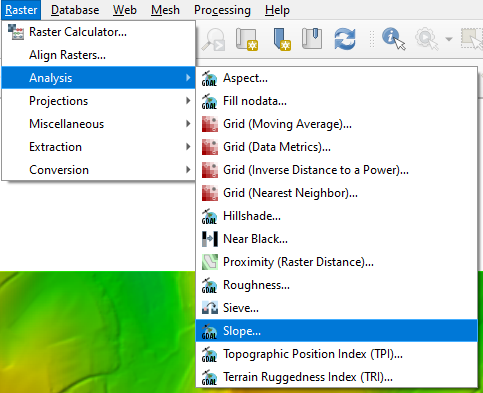2. In the Slope dialogue choose dtm_voidfilled as Input layer. Check the box to use the ZevenbergenThorne formula instead of Horn's one. The ZevenbergenThorne formula works better with less relief, while we use Horn's formula for steep areas. Choose slopedegrees.tif as output slope layer.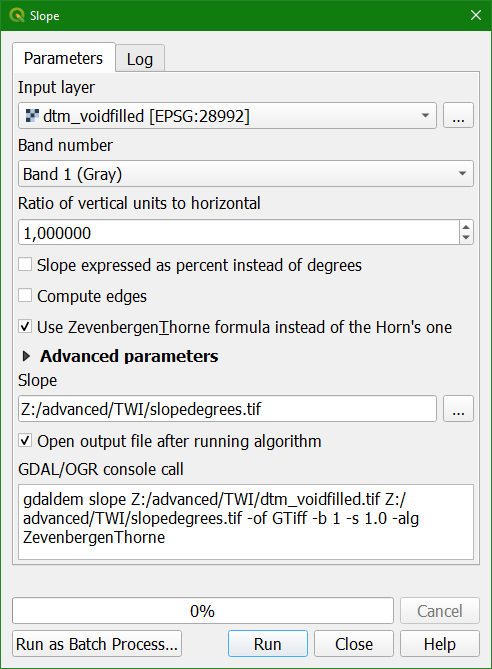3. Click Run. Close the dialogue after processing.

Because later we're going to calculate the natural logarithm (ln) we need to check if there are pixels with a slope of 0 degrees.

4. Click right on slopedegrees and choose Properties.

5. In the Layer Properties dialogue go to the Information tab and check the minumum value (STATISTICS_MINIMUM).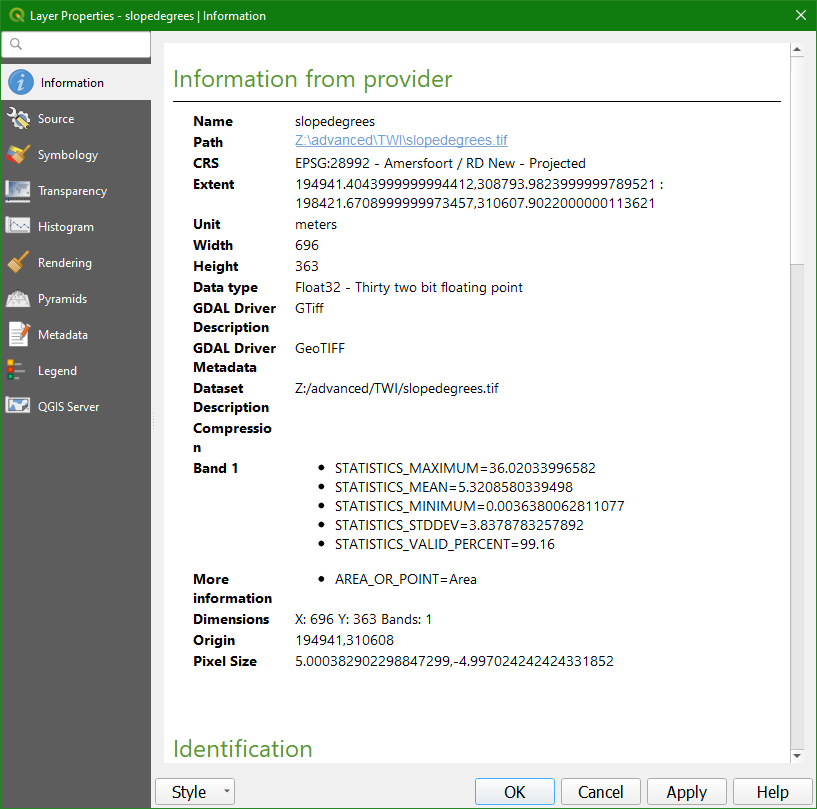In this case the minimum slope is larger than 0, so we can proceed. However, if the minumum slope is 0 you need to do the following steps, that you can also apply to this data, because it will not have any effect.

6. Click Cancel to close the Layer Properties dialogue.

7. In the main menu go to Raster | Raster Calculator.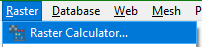8. In the Raster Calculator create the following equation:

 ( "slopedegrees@1" <= 0 ) * 1 + ( "slopedegrees@1" > 0 ) * "slopedegrees@1"

This means: if cells have a slope less than or equal to 0, then give value 1 (True) and multiply by 1. For the cells that are larger than 0, assing value 1 (True) and multiply with its slope value. The result will be 1 for cells <= 0 and the original slope for cells > 0.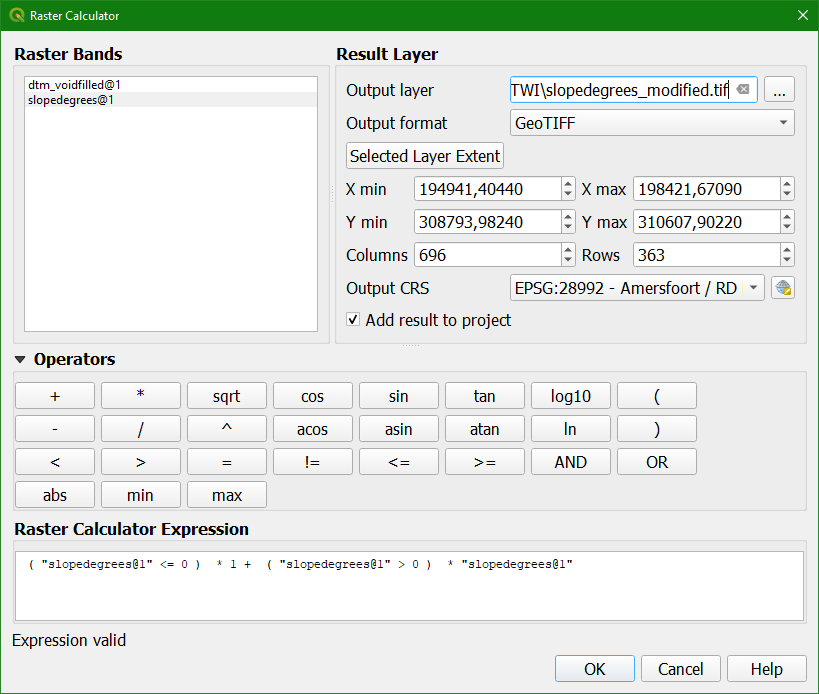9. Save the result to slopedegrees_modified.tif and click OK.

To convert from degrees to radians we need to multiply the pixel values by 0.01745

10. Go back to the Raster Calculator and calculate the following equation:

"slopedegrees_modified@1" * 0.01745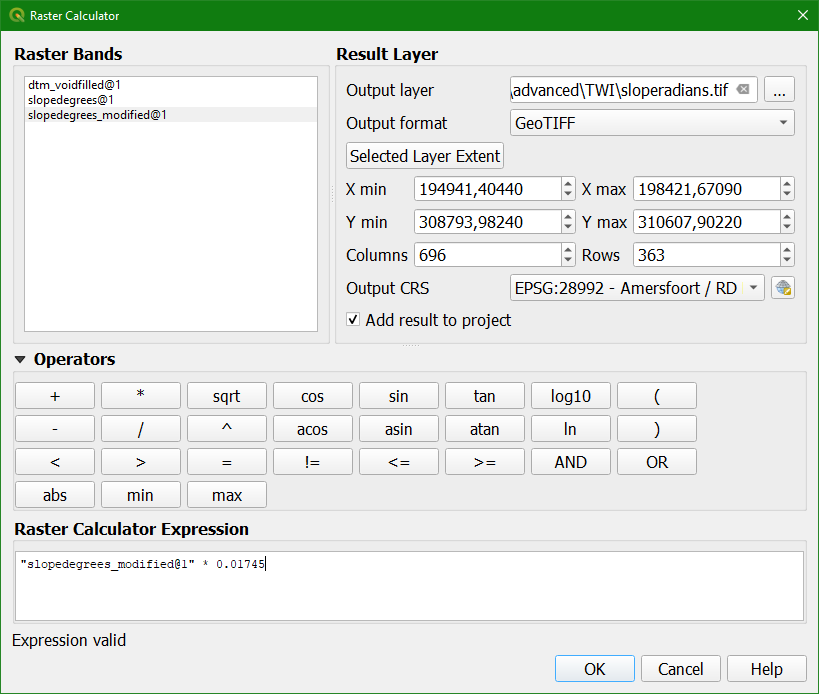11. Save the result to sloperadians.tif and click OK.

12. Style the result from yellow to red.

13. remove the other slope layers.